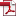• KSII Transactions on Internet and Information Systems
Monthly Online Journal (eISSN: 1976-7277)

# CA Joint Resource Allocation Algorithm Based on QoE Weight

Vol. 12, No. 5, May 30, 201810.3837/tiis.2018.05.018 , Download Paper (Free):#### Abstract

For the problem of cross-layer joint resource allocation (JRA) in the Long-Term Evolution (LTE)-Advanced standard using carrier aggregation (CA) technology, it is difficult to obtain the optimal resource allocation scheme. This paper proposes a joint resource allocation algorithm based on the weights of user’s average quality of experience (JRA-WQOE). In contrast to prevalent algorithms, the proposed method can satisfy the carrier aggregation abilities of different users and consider user fairness. An optimization model is established by considering the user quality of experience (QoE) with the aim of maximizing the total user rate. In this model, user QoE is quantified by the mean opinion score (MOS) model, where the average MOS value of users is defined as the weight factor of the optimization model. The JRA-WQOE algorithm consists of the iteration of two algorithms, a component carrier (CC) and resource block (RB) allocation algorithm called DABC-CCRBA and a subgradient power allocation algorithm called SPA. The former is used to dynamically allocate CC and RB for users with different carrier aggregation capacities, and the latter, which is based on the Lagrangian dual method, is used to optimize the power allocation process. Simulation results showed that the proposed JRA-WQOE algorithm has low computational complexity and fast convergence. Compared with existing algorithms, it affords obvious advantages such as improving the average throughput and fairness to users. With varying numbers of users and signal-to-noise ratios (SNRs), the proposed algorithm achieved higher average QoE values than prevalent algorithms.